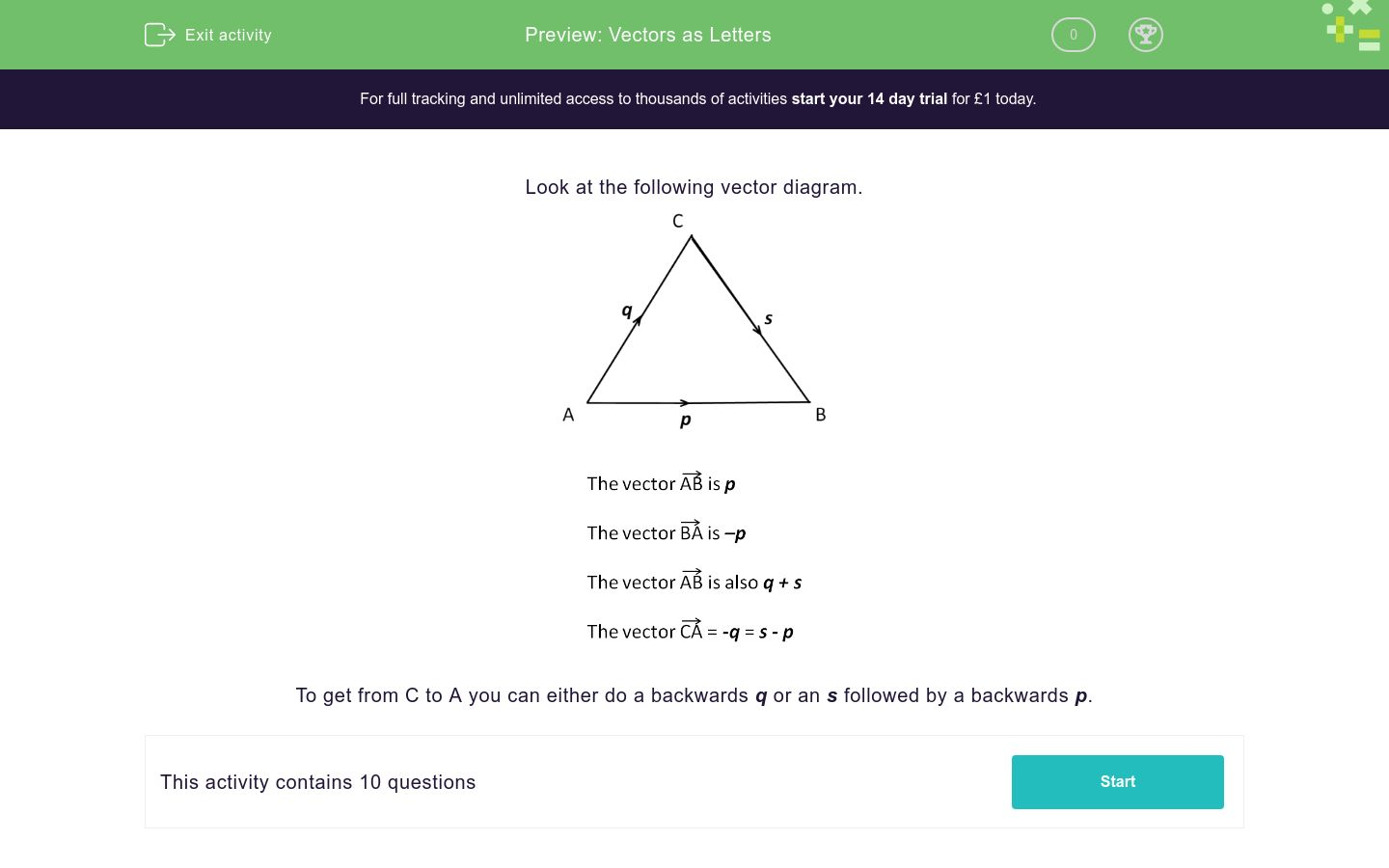# Vectors as Letters

In this worksheet, students must use letter notation to answer questions on vectors.Key stage:  KS 4

Curriculum topic:  Geometry and Measures

Difficulty level:### QUESTION 1 of 10

Look at the following vector diagram.To get from C to A you can either do a backwards q or an s followed by a backwards p.q + r

q - r

p - sq + r

p

q + r + sq + r

- q - r

s - pq - p

- p + q

-s - r

ABCD is a parallelogram.p

q

p + q

ABCD is a parallelogram.q - p

- p + q

p + q

ABCD is a parallelogram.q - p

- p + q

p + q

ABCD is a parallelogram.q - p

- p - q

-q - pr - s

t + u

t - us

r + u + t

r - u - t

r + u - t

u - t + r

• Question 1q + r
p - s
EDDIE SAYS
q followed by r
p followed by a backwards s
• Question 2p
q + r + s
EDDIE SAYS
p
q followed by r followed by s
• Question 3- q - r
s - p
EDDIE SAYS
A backwards q followed by a backwards r
s followed by a backwards p
• Question 4q - p
- p + q
-s - r
EDDIE SAYS
A backwards p followed by q (order doesn't matter)
A backwards p followed by q
A backwards s followed by a backwards r
• Question 5

ABCD is a parallelogram.q
EDDIE SAYS
This is simply the same as going from A to D as ABCD is a parallelogram.
• Question 6

ABCD is a parallelogram.p + q
EDDIE SAYS
p followed by q
• Question 7

ABCD is a parallelogram.q - p
- p + q
EDDIE SAYS
q followed by a backwards p
A backwards p followed by q
• Question 8

ABCD is a parallelogram.- p - q
-q - p
EDDIE SAYS
A backwards p followed by a backwards q
A backwards q followed by a backwards p
• Question 9r - s
t - u
EDDIE SAYS
A backwards s followed by an r
t followed by a backwards u
• Question 10s
r + u - t
u - t + r
EDDIE SAYS
Simply s
r followed by u followed by a backwards t
The last option is the same but in a different order.
---- OR ----

Sign up for a £1 trial so you can track and measure your child's progress on this activity.

### What is EdPlace?

We're your National Curriculum aligned online education content provider helping each child succeed in English, maths and science from year 1 to GCSE. With an EdPlace account you’ll be able to track and measure progress, helping each child achieve their best. We build confidence and attainment by personalising each child’s learning at a level that suits them.

Start your £1 trial